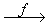Home MonkeyNotes Printable Notes Digital Library Study Guides Study Smart Parents Tips College Planning Test Prep Fun Zone Help / FAQ How to Cite New Title Request

CHAPTER 1 : FUNCTIONS

1.1 Functions And Mapping

Definition: Let A and B be any two non-empty sets. Let A = {p, q, r} and B = {a, b, c, d}. Suppose by some rule or other, we assign to each element of A a unique element of B. Let p be associated to a, q be associated to b, r be associated to c etc. The set {(p, a), (q, b), (r, c) } is called a function from set A to set B. If we denote this set by f then we write f : A ® B which is read as "f " is a function of A to B or f is a mapping from A to B.

Definition: Let A and B be two non-empty sets. Suppose there exists a rule of correspondence, denoted by f which associates to each member of A, a unique member of B. Then f is called a function or a mapping from set A to set B.

It is denoted by f : A ® B or ABYour browser does not support the IFRAME tag.

Note that :

1. The set A is called "domain" of 'f '

2. The set B is called "codomain" of 'f '

3. The element y Î B which the function 'f' associates to the element x Î A is denoted by f(x) i.e.

y = f(x) and y is called the 'f ' image of x or value of ' f ' at x. The element x is also called as pre-image of y. Every element of A has a unique image but each element of B need not have as the image of an element in A. There can be more than one element of A which has the same image in B.

4. The set of these images in B is called the range of ' f ' i.e. the range of a function as the images of its domain. We denote the range of f : A ® B by f (A). Thus f (A) = { f (x) : x Î A } Þ f (A) Í B.

5. If A and B are any two non-empty sets, then a function f from A to B is a subset f of A ´ B satisfying the following conditions:

(i)x Î A, (x ,y) Î f for some y Î B

(ii) (x , y) Î f and (x, y1) Î f Þ y = y1

The first condition ensures that there is a rule which assigns to each element x Î A some element y Î B. Thus each element in A will have an image. The second condition guarantees that the image is unique.

In other words, a function is a set of ordered pair (x, y), such that for each first element x, there corresponds a unique element y.

6. The domain variable is often referred as the independent variable and the range variable is referred as the dependent variable.

Index

Introduction

1.1 Functions And Mapping
1.2 Functions, Their Graphs and Classification
1.3 Rules for Drawing the Graph of a Curve
1.4 Classification of Functions
1.5 Standard Forms for the equation of a straight line
1.6 Circular Function and Trigonometry

Chapter 2